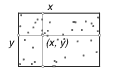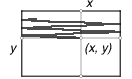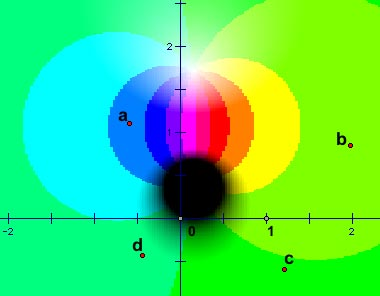# Graphing Planar Transformations as Surface Plots

Despite the appeal of dragging, Figure 6 reveals one of the shortcomings of the conventional solution to the "problem of complex graphing." When both pre-image (the circle) and image (the origin-winding quasi-cardioid) are superimposed on the same coordinate system, legibility suffers. In more involved visualizations, it becomes increasingly difficult to distinguish input from output, "cause" from "effect." More generally, the conventional approach struggles between opposing imperatives. On the one hand, we want our image and pre-image to be as large as possible, since we are interested in gaining a sense of the effect of our complex transformations across the entire plane, not just on a single point or point locality. On the other hand, we are interested in keeping our image and pre-image small, to avoid overlap. Physically isolating the image into a separate visualization from the pre-image (a separate illustration of the plane) is one approach, but replaces the immediacy of a unified visualization with a more abstract representation requiring additional interpretation to reconcile conceptually. Thus we are encouraged to move beyond the conventional solution, and to explore techniques for visualizing the effect of a complex transformation over the entire plane, on the entire plane.

To proceed in this direction, we need first to establish techniques for plotting surfaces, and since we are in a dynamic geometry environment, we must derive these from mathematical principles and primitives rather than look to built-in software commands. To plot a surface ideally, one needs first to be able to visit every point on the plane. Practically, we seek to visit some regular subset of points in a planar region. Once we can visit such points, we can evaluate a condition or function there, to determine the value of the surface at or above that planar point. But traditional loci or function plots in Sketchpad and loci in Cabri are one-dimensional curves, rather than two-dimensional areas. So this basic requirement—a tour of some planar region—is sufficiently challenging from the perspective of compass-and-straightedge constructions, or even from the much richer set of primitives that characterize a dynamic geometry environment, that it's worth struggling with, with students. Workable solutions draw on a variety of mathematical approaches and governing metaphors:

Space filling curves. Can a one-dimensional curve be wrapped in such a way that it fills a two-dimensional area? If so, sampling points on the curve—that is, taking a locus of a point traveling that curve—is equivalent to sampling a planar region. As well as wrapping configurations, one can imagine torn-and-reassembled configurations, as when one tears a (near) one-dimensional roll of wallpaper into strips that, reassembled side-by-side, cover a two-dimensional wall.Random motion. At right, x and y are points on their respective segments; (x, y) is their projection. Randomly varying x and y along their segments causes (x, y) to sample randomly the rectangular region.Synchronized motions. If x and y tour their segments at different rates, their projection scans the rectangle in much the way a cathode ray "scans" the phosphor screen of a conventional television monitor. Different speeds can be achieved with Sketchpad's animation, or the velocity of each particle can be constructed as a separate parameterization of time.Iterative decomposition. In this approach, the quadrilateral ABCD is decomposed (via Sketchpad's Iterate command) into its four corner quadrilaterals. Repeating this decomposition recursively a few times generates progressively smaller-meshed "screens" covering the original quadrilateral area (with 4, 16, 64, 256, 1024… interior samples). The advantage of this approach is its variability of resolution—one can work at low resolution when building a draft model for speed and convenience, and then increase the depth of iteration to improve the sampling detail of a final visualization.Once a technique has been established for sampling points on the plane—that is, for visiting a region of complex values—surface plots of complex functions or transformations can be built by evaluating the function at each sampled point, and characterizing the surface at that point by the resulting value. The particular geometric interpretation of that value can vary richly, as the visualizations of Figure 8 attempt to suggest.Figure 8a. Vector field of f(z) = az + b.

In Figure 8a, an arrow connects each sampled point z with its image f(z)—quite literally, z goes to f(z). Another possible vector plot displays f(z) as a vector quantity translated to the tip of z. (In such visualizations, roots display no arrows, rather than display arrows pointing to the origin.)Figure 8b. Farris plot of the general
Mobius transformation f(z) = (az + b)/(cz + d).

Figure 8b uses Sketchpad's parametric coloration to present a dynamic version of a planar coloring plot proposed by Frank Farris (1998) in his MAA review of Needham (1997). In this approach, a standard colorization is given to the complex plane, in which the hue of some point z corresponds to its argument (its angle with respect to the positive real axis) and the shade value or tonal density ranges with z's modulus (distance from the origin), from white at the origin to black at infinity. Given this or any other distinctive coloration of the plane, a Farris plot of the complex function f involves recoloring the plane so that each point z has the color assigned to point f(z) in the canonical coloration. These vivid plots readily reveal algebraic multiplicities through multiple-spiraling hue configurations, and the roots and poles of f appear as pure white and pure black locations.Figure 8c. Modular surface
of f(z) = a/(b + z2).

Finally, Figure 8c depicts perhaps the most literal interpretation of "surface plotting." Here the height of the visual surface corresponds to the modulus of the given function. These modular surfaces reach down to touch the plane at their roots, and explode upwards toward infinity at their poles.